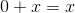# Pre-Algebra : Additive Identity Property

## Example Questions

### Example Question #1 : Additive Identity Property

Which statement demonatrates the additive identity property?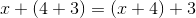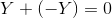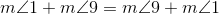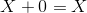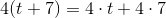Explanation:

By the additive identity property, zero added to a number yields that second number as the sum. This is shown by the statement.

### Example Question #47 : Identities And Properties

Which of the following demonstrates the additive identity property?

If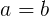, then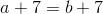.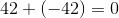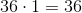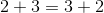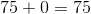Explanation:

Pre-algebra brings with it many different properties for memorization, and it is easy to forget one or mix two of them up.  But maybe we can jog our memory by looking closely at the name of the property.  The first word in the additive identity property is "additive".  This tells us very quickly that the property involves addition.  That means we can already eliminate any answer choices that don't involve adding, which in our case is the choice.

With four choices left, we look at the next word in the name, "identity".  The identity of something is what the thing is.  In order for a spy to avoid being caught, he/she might change their idenity.  They might take a different name, wear a wig, fake an accent, or dress differently.  If the value of a number changes, its identity changes.  In math, the only number I can add to any number without changing its value is 0.  Therefore, we call 0 the additive identity because adding it preserves the identity of a number.  This fact--namely that adding 0 to a number results in the same number--is what we call the Additive Identity Property.

If we look once more at our answer choices, the only one involving the addition of 0 to a number is the choice.  This is the correct answer.

### Example Question #48 : Identities And Properties

Which of the following statements demonstrates the identity property of addition?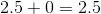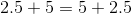None of the examples in the other responses demonstrates the identity property of addition.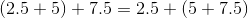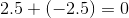Explanation:

The identity property of addition states that there is a number 0, called the additive identity, that can be added to any number to yield that number as the sum. Of the four statements,demonstrates this property.

### Example Question #49 : Identities And Properties

Which of the following best represents the additive identity property?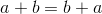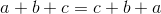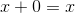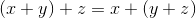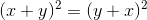Explanation:

The additive identity property states that adding zero to any value will leave the value unchanged.  The equations that will best describe this scenario is: